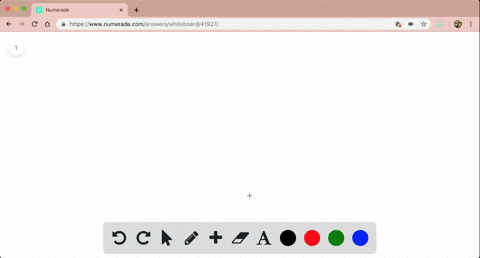🎉 The Study-to-Win Winning Ticket number has been announced! Go to your Tickets dashboard to see if you won! 🎉View Winning Ticket## Discussion

You must be signed in to discuss.

## Video Transcript

So today we will be solving the integral from 2 to 6 of either the two x plus three y d X. Now the first thing we do when we look at this is we we can figure out howto integrate e to the X. Something simpler. So we want to use what's called u substitution. And to do that, we have to pick what's Are you gonna be So are you in this case is going to equal to X plus three. Why? And that means that our d'you has to equal, um, two times DX because three wise like a constant and so the derivative of that in terms of X will be zero. So now I have my you and might do so I can rewrite my integral here, and I'm going to write just a definite interval and then we'll bring back the two in the six. So this will be the mineral of E to the u times D'You And don't forget about this two over here, we have to make ah, 1/2 and I'm gonna bring this in the front. So since it's a constant, we can take it out, and it could be 1/2 times this interval of E to the U D U. So now we know how to integrate this because the inter role of either the U is just eat of the U. Um, if you don't remember this, it's also the interval of or it's e to the you and then over natural log of your the base. But the natural log of ease Just one. And so that's why he is a special function where when we integrate this, we just get 1/2 e to the U. Normally, if we were just solving the definite and a role we would put plus C at the end. But we don't need that here because we're gonna end up plugging in this, too. In this six sow, the seed would cancel. So I'm gonna write 1/2 e to the you, which I'm gonna replace my you back with two X plus three. Why? So say two X plus three. Why? And I'm gonna solve this from 2 to 6 or integrate this rate from from 2 to 6. So first, what we're gonna do is plug in the six for X. Okay, so we're gonna get 1/2 times E to the to 10 6 which is 12 plus three. Why and then minus. And now I plug in the two. So I have 1/2 again times E to the two times two, which is four plus three wives. And now we can write this a little bit nicer just by writing the 1/2 once. And you could have done this to start. But now we'll have 1/2 and then we'll do e to the 12 plus three. Why minus e to the four plus 31 And this will be our answer for our indefinite integral above right here.